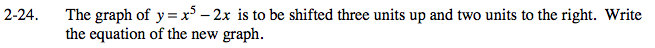### Home > PC > Chapter 2 > Lesson 2.1.2 > Problem2-24

2-24.

The graph of y = x5 − 2x is to be shifted three units up and two units to the right. Write the equation of the new graph. Homework Help ✎Move the graph to the right 2 units.

y = (x − 2)5 − 2(x − 2)

Shift the graph up 3.

y = (x − 2)5 − 2(x − 2) + 3